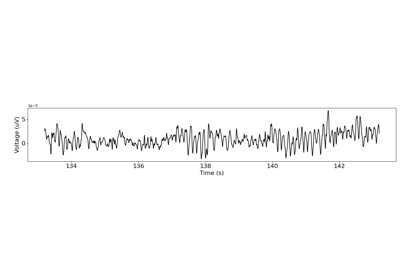# neurodsp.spectral.trim_spectrum¶

neurodsp.spectral.trim_spectrum(freqs, power_spectra, f_range)[source]

Extract a frequency range of interest from power spectra.

Parameters
freqs1d array

Frequency values for the power spectrum.

power_spectra1d or 2d array

Power spectral density values. If 2d, should be as [n_spectra, n_freqs].

f_range: list of [float, float]

Frequency range to restrict to, as [f_low, f_high].

Returns
freqs_ext1d array

Extracted frequency values for the power spectrum.

power_spectra_ext1d or 2d array

Extracted power spectral density values.

Notes

This function extracts frequency ranges >= f_low and <= f_high. It does not round to below or above f_low and f_high, respectively.

Examples

Trim the power spectrum of a simulated time series:

>>> from neurodsp.sim import sim_combined
>>> from neurodsp.spectral import compute_spectrum
>>> sig = sim_combined(n_seconds=10, fs=500,
...                    components={'sim_powerlaw': {}, 'sim_oscillation' : {'freq': 10}})
>>> freqs, spectrum = compute_spectrum(sig, fs=500)
>>> freqs_ext, spec_ext = trim_spectrum(freqs, spectrum, [1, 30])


## Examples using neurodsp.spectral.trim_spectrum¶